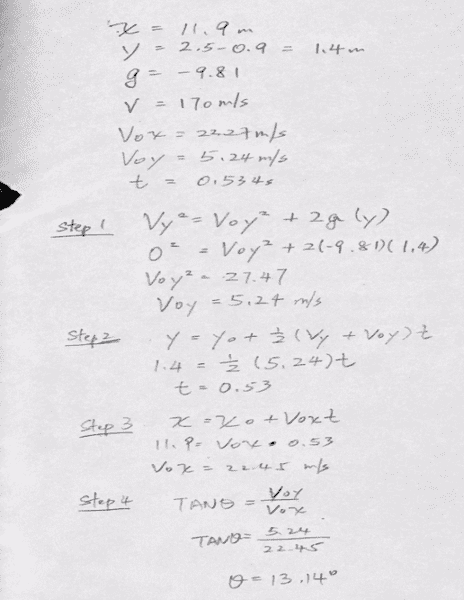# 2D kinematic problem: Tennis serve

## Homework Statement

Serving at a speed of 170 km/h a tennis player hits the ball at a height of 2.5 m and an angle θ below the horizontal. The service line is 11.9 m from the net, which is 0.91 m high. What is the angle θ such that the ball just crosses the net? Will the ball land in the service box, whose out line is 6.40 m from the net? [ANS: 6. 1°]

Vy2=Voy2-g(y-y0)
y=y
0+½(Vy+V0y)t
x=x0+V0xt

## The Attempt at a Solution

http://blob:https://www.physicsforums.com/e28d4bae-4e89-4ed3-a5bb-353215f729f5 [Broken][/B]
Please let me know what is the problem in the solution.
i don't know how to find the answer [6.1°]

Last edited by a moderator:

lewando
Homework Helper
Gold Member
v = 170 km/h or m/s?

how did you get t=0.534s? as far as I can tell, time is unknown?

you need to convert 170km/h into m/s.

from step 1, it looks like you've made the assumption that vfy=0, but there is no reason to think that the ball would have 0 velocity by the time it got to the net or floor.
Did you post a second picture below the first one that shows step 1? If you did, it's not showing, perhaps try posting it again.

Last edited:
v = 170 km/h or m/s?
yes , i made a mistake , it is 170km/h

how did you get t=0.534s? as far as I can tell, time is unknown?

you need to convert 170km/h into m/s.

from step 1, it looks like you've made the assumption that vfy=0, but there is no reason to think that the ball would have 0 velocity by the time it got to the net or floor.
Did you post a second picture below the first one that shows step 1? If you did, it's not showing, perhaps try posting it again.
the given has X=11.9m /Y=1.4m / V=170km/h g=-9.81
i don't know how can find y0y or t
i didn't, just one picture

The question is asking us to solve for θ.
First I would convert the velocity 170km/h to m/s, then resolve this into its horizontal and vertical components. They will be in terms of θ.
Then use kinematic equations and the given information to derive an equation in terms of θ. Then the plan is to solve for θ.

Last edited:
haruspex
Homework Helper
Gold Member
2020 Award
Y=1.4m
Is that supposed to be 2.5m-0.91m?

I'm wondering about this question also. It's a question from the OpenStax AP Physics textbook. I have two equations with two unknowns (theta and t) and I'm not sure how to proceed.

haruspex
Homework Helper
Gold Member
2020 Award
I'm wondering about this question also. It's a question from the OpenStax AP Physics textbook. I have two equations with two unknowns (theta and t) and I'm not sure how to proceed.
I can neither verify your equations nor suggest how to proceed without seeing them.

The initial velocity is 47.2 m/s

y-component: $$0.91 = 2.5 - 47.2*sin(\theta)*t - 4.9*t^2$$

x-component: $$11.9 = 47.2*cos(\theta)*t$$

So, we have two equations and two unknowns (theta and t), but I don't see how to proceed from here.
I would appreciate any help. Thanks a lot.

haruspex
Homework Helper
Gold Member
2020 Award
The initial velocity is 47.2 m/s

y-component: $$0.91 = 2.5 - 47.2*sin(\theta)*t - 4.9*t^2$$

x-component: $$11.9 = 47.2*cos(\theta)*t$$

So, we have two equations and two unknowns (theta and t), but I don't see how to proceed from here.
I would appreciate any help. Thanks a lot.
You can use the standard procedure for solving simultaneous equations: use one equation to express one unknown in terms of the other and use that to substitute for that first unknown in the other equation.
You have two equations to choose from for that first step, and two choices of which way to do the substitution. One of the four choices is easier than the others.

$$t = \frac{11.9}{47.2*cos(\theta)}$$

$$0.91 = 2.5 - 11.9*tan(\theta) - \frac{4.9*(11.9)^2}{(47.2*cos(\theta))^2}$$

But I'm not sure how to solve the final equation for theta.

haruspex
Homework Helper
Gold Member
2020 Award

$$t = \frac{11.9}{47.2*cos(\theta)}$$

$$0.91 = 2.5 - 11.9*tan(\theta) - \frac{4.9*(11.9)^2}{(47.2*cos(\theta))^2}$$

But I'm not sure how to solve the final equation for theta.
Good choices.
Next, it helps to know the quadratic relationship between tan and sec.

Oh, thanks for pointing this out. Then I think I can put the equation in this form:

$$a*tan^2(\theta) + b*tan(\theta) + c = 0$$

Then I would use the quadratic formula to solve for tan(theta)?
Is this the standard way to solve a question like this?
Is there not a simpler way to find theta and time t?

In any case, thanks a lot for your help.

haruspex